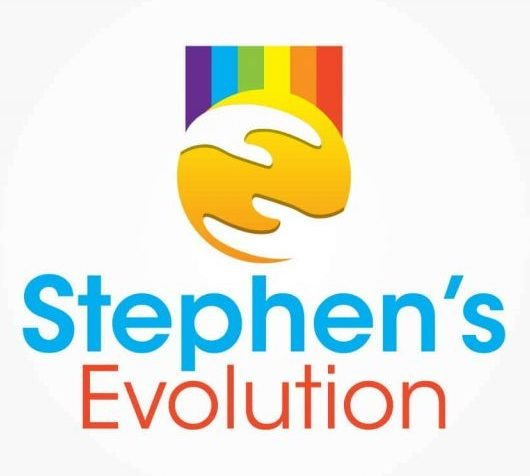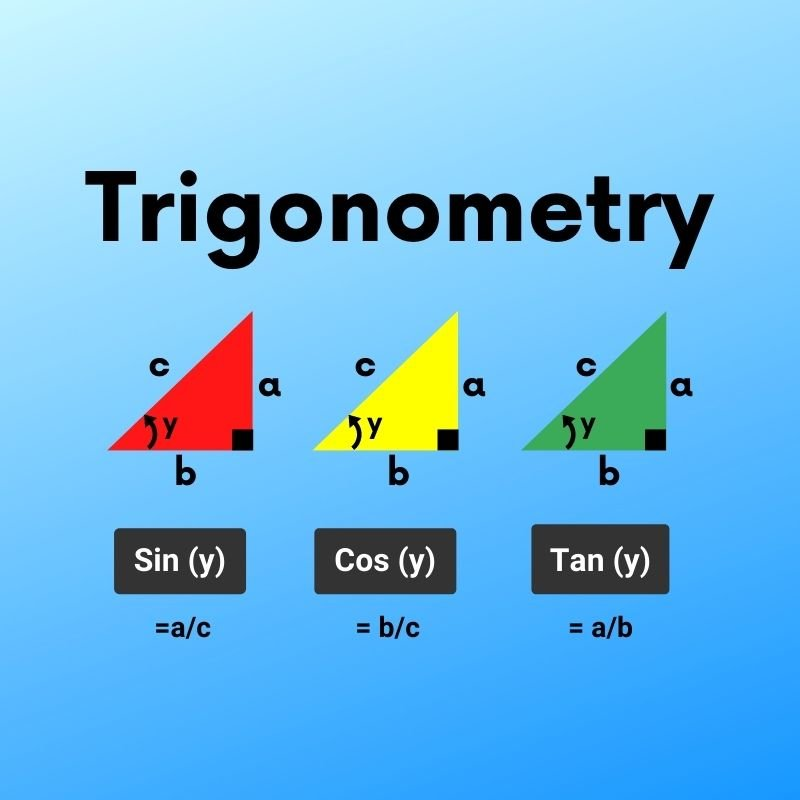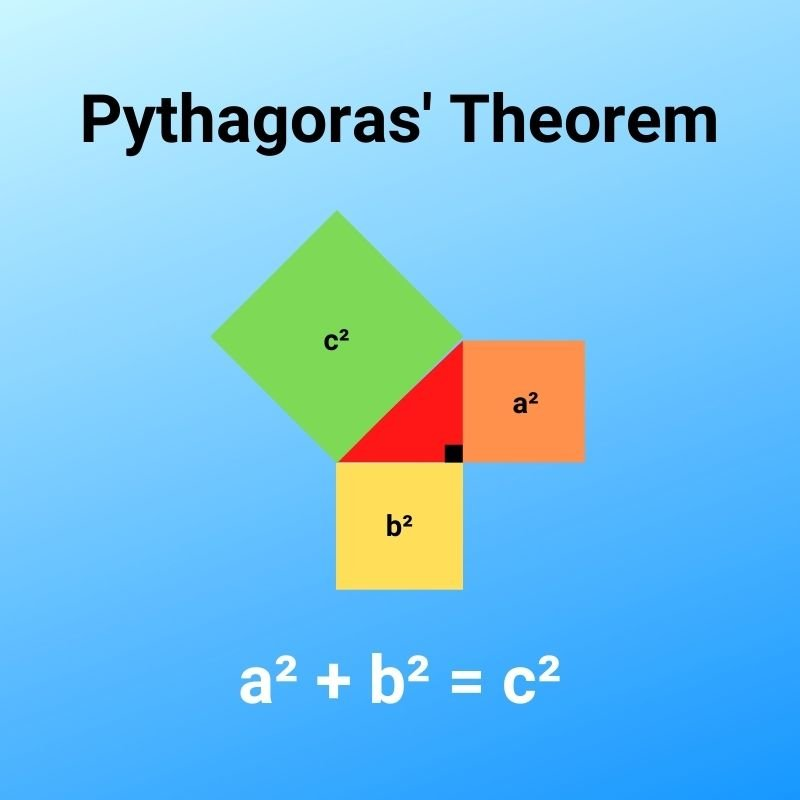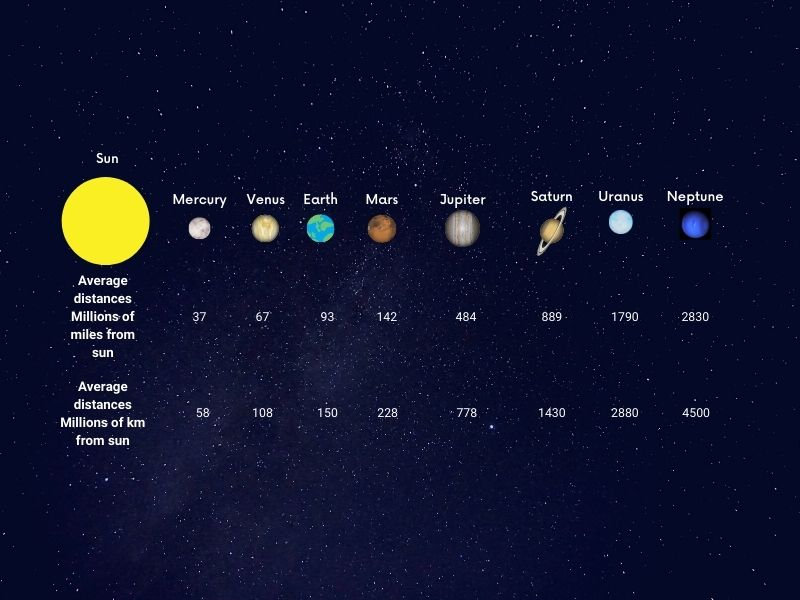# Ways to support maths students with autism aged 11 to 16

Stephen McHugh
This post was last updated on
August 19, 2022
Category :

Mathematics was another subject I began to excel at during my life at secondary school, especially as my language skills and general understanding improved. This meant I was able to understand new mathematical concepts more easily. Below I talk about ways that were beneficial in supporting me when making progress in mathematics in senior school. You're welcome to use them for ideas to support autistic students at senior school in teaching them mathematics, whether you are a parent or in a teaching capacity.

Being interested in numbers lead to my interest in clocks, watches, stopwatches, and anything time-related. My interest in large numbers and extremities lead me to calculate the number of hours, minutes, and seconds in a number of days, weeks, months, and years.

Knowing the heartbeats on average 72 times per minute led me to do calculations regarding the number of beats over lifetimes.

72 × 60 = 4,320 beats per hour;

4320 × 24 = 103,680 beats per day;

103,680 × 365 = 37,843,200 beats;

37,843,200 × 80 = approximately 3,000 million beats over life time.

## Equations

For solving equations I’d read maths textbooks and follow techniques used to work out solutions similar to the equations I was working with. An example would be working out the value for ‘a’ below. The idea is to get ‘a’ on one side equaling a number on the other side.

5a + 7 = 3a + 13

2a + 7 = 13 (Take 3a, which is the lower value involving ‘a’ from both sides)

2a = 6 (Take 7, which is the smaller of the two integers, from both sides)

a  = 3 (Divide 6 by 2)

Note that 5a = a + a + a + a + a ; a = 1a

G =  H - TS. Make S the subject of this formula.  (TS = 1TS; 2TS = TS + TS; G = 1G; H=1H)

One method I liked here was to use my own values for letters in equations to verify my solution. You could let G = 100, H = 150, T = 10 and S = 5.

100 = 150 - (10 x 5)

TS = H - G (Add TS to and take G from both sides) 10 x 5 = 150 - 100

S = (H - G)/ T (Divide both sides by T; When you do TS/T, both T’s cancel, leaving S)

5 = (150 - 100)/10

## TrigonometryTrigonometry is a branch of mathematics where one can use functions known as sin, cos, and tan when calculating angles and the lengths of sides in triangles. This topic of mathematics I quickly felt at home with and found interesting. Our teacher informed us it was a challenging topic worth a lot of marks, which fueled my determination to master it. The SOH CAH TOA is an interesting saying and a tip our maths teacher at high school introduced us to. To me, it is a useful way of helping us to remember which sides of a right-angled triangle are related to whatever trigonometric function.

Picture a right-angled triangle. A right angle is 90 degrees. One side is called an opposite(O) because the angle concerned is facing it. The adjacent side is so-called because it is adjacent(A) to the angle in question. The longest side of a right-angled triangle is called the hypotenuse(H). The letter ‘x’  in each case below indicates the value of the angle.

Sin: Sin(x) = O/H; Inv Sin (O/H) = x; O = A × Sin(x) H = O divided by Sin(x)

Cos: Cos(x) = A/H; Inv Cos (A/H) = x; A = H × Cos(x); H = A divided by Cos(x)

Tan: Tan(x) =O/A; Inv Tan (O/A) = x; O = A × Tan(x); A = O divided by Tan(x)

## Pythagoras's theoremAnother topic I readily mastered in year 9 was Pythagoras’s theorem. This involves calculating the lengths of sides of right-angled triangles using squared values of the length of the sides. To work out the length of the longest side (hypotenuse), let’s suppose the shorter sides were 3 and 4. The patterns I recognised are outlined below.

• Square both 3 and 4 to give 9 and 16 respectively.
• Add the values 9 and 16 to give 25,
• Square root 25 to give the length 5.

Suppose you had the values of the hypotenuse and one of the shorter sides.

• 5² = 25,
• 3² = 9.
• Take 9 from 25 gives 16
• The square root of 16 gives 4.

C is the hypotenuse and A and B are the other two shorter sides. So, to sum up

A²  + B² = C²

C² - B² = A²

C² - A² = B²

## Personal interestsPersonal interests can play important roles in our learning. Whenever I did personal space projects, I wanted to calculate the speed of the orbits of the planets around the sun. To do that, I had to work out the circumference of the orbits of the planets around the sun. Planets can be considered to go round in the circle around the sun at constant speeds.

I knew the distances of a particular planet from the sun to be a radius. Multiplying the radius by 2 gives the diameter of the orbit.  Eventually I found out that the circumference of a circle could be worked out by doing the following calculation, which is the diameter of the orbit × π(3.142).

Let's take the Earth for example;

93 million miles(orbit radius)  × 2 = 186 million miles(orbit diameter);

365 days in a year × 24 hours per day = 8760 hours per year;

186 million * 3.142 = approximately 584.4 million

584.4 million / 8760 = approximately 66,700 mph (approximately 107,000 kph)

This gave me an insight into counting circumferences from the radius and diameter of circles.

## Rounding numbers

When it came to rounding numbers we were taught to round a number ending with a 5, 6, 7, 8, or 9 up. Examples here are 78 which, being rounded to the nearest ten is 80, and 45 to 50. For numbers ending with a 1, 2, 3, or 4, we round them down, e.g. 33 rounded to the nearest ten is 30. The methodology shown to us here was easy for me to apply to questions related to rounding.

## Simultaneous equations

My grade C in GCSE maths in year 10, is one achievement I still look upon very favourably. The reason for this was that I struggled to understand new concepts and apply new knowledge in primary school. That gave me the confidence that my language skills and knowledge application were improving, despite the fact I still had some way to go. Despite this, I was still able to achieve a good GCSE grade.

As well as after-school maths lessons, I had private lessons locally, which continued into and throughout year 11. One session at my private lessons outside of school proved beneficial to me when learning how to do simultaneous equations. I was taken patiently and painlessly through a step-by-step process outlined below readily understood by me at the time.

1. 3x + 2y = 7
2. 5x - 3y = 37

Multiply equation 1 by 5 using 5 from the 5x in 2. (An alternative could be to multiply eq 1 by using -3 from -3y in equation 2. But for me it’s easier using the positive value).

Multiply equation 2 by 3 using 3 from the 3x in 1. (The other option here could be to multiply eq 2 by using 2 from +2y in equation 1).

1. 15x + 10y = 35
2. 15x - 9y = 111
• You can now take away either 2 from 1 or 1 from 2.
• We’ll now take away 2 from 1 to leave us with just 19y = -76 ( 19y comes from 10y - (-9y) = 10y + 9y = 19y).
• The next step is -76/19 to obtain -4.
• The final step is to substitute -4 into a y into one of the equations. Let’s do that in equation 1.

3x + 2(-4) = 7

3x - 8 = 7

3x = 7 + 8

x = 15/3 = 5

## Factorisation of and multiplying out equations

With the lessons I had locally, here's another method shown which benefited me. It involved factorising equations. I was taught to look for common factors, which go outside brackets.

For ab + a  a is seen to be the common factor. Factorising it gives us a(b+1).

For ab + bc  b is seen to be the common factor. Factorising it gives us b(a+c).

For abπ + acπ aπ is seen to be the common factor. Factorising it give us aπ(b+c).

(a + 2)(a - 3) can be multiplied out using the following method.

a +2
a +2a
-3 -3a -6

a × a = a²; a × 2 = 2a; -3 × a = -3a; -3 × 2 = -6. The power of 2 used for a² indicates that a is used twice in a multiplication operation;
2a and -3a give us -a. Here use 2 and -3 in this number operation because both numbers use the same letter, and they have the same power.

The letter a is used once in each multiplication operation 2 × a = 2a; -3 × a = -3a.

We see that a² is on its own, as is -6. So, we see the final result of the equation multiplied out below.

a² +2a - 3a - 6 = a²-a-6. This is known as a quadratic equation.

## Percentage increases and decreases

Percentage is represented by the sign %. It involves numbers being expressed as fractions of 100. Take a look at the following examples below. The methods used for them were easy for me to master.

You have £10,000 to put in a savings account. The savings account has an interest rate which enables an increase of 5% per year. You have to leave the money in the account for 5 years. How much will it be worth after this time?

100% + 5% = 105%; 105/100 = 1.05.

10,000 × 1.05 × 1.05 × 1.05 × 1.05 × 1.05 = 10,000 × (1.05^5) = 12762.82 (2dp)

You buy a car brand new worth about £15,000. After one year it has lost 20% of that value. What is it worth then?

100% - 20% = 80%; 80/100 = 0.80

£15,000 × 0.80 = £12,000

## Conclusion

Mathematics is the study of numbers and related operations. There are many concepts of mathematics which are applied to situations involving numerical operations in everyday life, such as engineering, calculating costs, and many other situations besides.  From my experiences, those of us with autism and language development delays experience difficulties and delays with mastering new concepts and applying them to everyday problems in primary school. See a link below to an earlier post in which I describe what helped me with maths during my primary school days.

Ways you can teach maths to children with autism

Therefore, for me, gaining a grade C a year earlier than typical GCSE students is one of the highlights of my life. With the right approaches, support and guidance, progress can be made and good GCSE grades can be gained, including doing work in relation to one's special interests and any facts they may find particularly fascinating.

If you're on the autism spectrum, and experienced language development delays, what approaches helped you to understand mathematical concepts more easily?

And if you're in a teaching capacity, or were, what methods did you use to support any autistic students? Let me know in the comments section below.